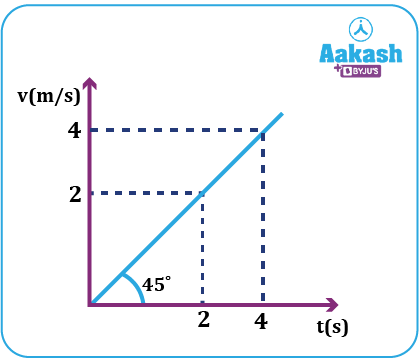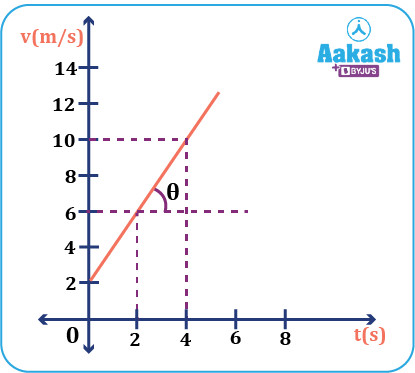• Call Now

1800-102-2727•

# Difference between Acceleration and Velocity - Practice problems, FAQs

Sachin drives to work everyday in his scooty. One day, he notices a KTM race past him. In order to catch up with him, he increases his throttle. Now he finds himself alongside the KTM. During this instant, the reading on his speedometer had changed – he was initially riding at 40 km/hr, but now he finds that he is racing at 80 km/hr. Sachin then becomes tired of chasing and decides to slow down his scooty; so he applies the brakes–gradually slowing down and coming to rest. In other words, one can say that Sachin has accelerated in the first case–increasing his speed. On the other hand, while slowing down, he has decelerated. In this article, we will explore the differences between velocity and acceleration.

• What is velocity?
• What is instantaneous velocity?
• What is acceleration?
• Difference between velocity and acceleration
• Practice problems
• FAQs

## What is velocity?

Velocity is defined as the change in displacement divided by the time taken. It is a vector quantity. Velocity can be positive or negative. For instance, when a car is moving along the negative y- axis, its velocity is negative considering that the displacement of the car is negative.

The unit of velocity is m/s and its dimensional formula is $\left[L{T}^{-1}\right].$

## What is instantaneous velocity?

The velocity of a body at a certain instant of time is called instantaneous velocity (vinst). It signifies the instantaneous displacement rate with respect to time.

${v}_{inst}=\frac{dx}{dt}$

$\frac{dx}{dt}$ denotes the differentiation of displacement with respect to time.

## What is acceleration?

Acceleration is the rate of change of velocity with respect to time. Mathematically, it can be expressed as,

It is a vector quantity. Its unit in SI system is ms-2.

vf indicates the final velocity and vi indicates the initial velocity at time instants tf and ti respectively.

Slope of the graph plotted between velocity on the vertical axis and the time on the horizontal axis is the velocity-time graph. The slope of the tangent drawn to the velocity time graph gives acceleration.In the above figure, the acceleration between 2 to 4 sec can be calculated from the slope of the velocity time graph as,

Also, the slope=tan 450=1.

In differentiation form, acceleration can be written as $a=\frac{dv}{dt}$

Note:

1) When a body is accelerating in the rightward direction, its acceleration is positive. For example, if a bike speeds up from 2 ms-1 to 4 ms-1 in 2 s rightwards, we get acceleration

2) When a body is accelerating in the leftward direction, its acceleration is negative. For example, if a bike speeds up from -2 ms-1 to -4 ms-1 in 2 s, we get acceleration

Defining the direction is necessary. The direction for which we consider positive sign, the opposite of it, by default gets the negative sign.

## Difference between velocity and acceleration

 Velocity Acceleration It gives an idea about change in displacement per unit time. It gives an idea about change in velocity per unit time. Its unit is m/s. Its unit is m/s2. Dimensional formula is $\left[{LT}^{-1}\right]$ Dimensional formula is $\left[{LT}^{-2}\right]$

Video explanation

## Practice problems

Q. A particle initially moving towards the east with 50 m/s then takes left and then starts moving north with a speed of 50 m/s in an interval of 1 sec. What is the magnitude and direction of the average acceleration?

A. Considering the unit vector along the east as i and along the north as j,

given,

Direction of acceleration,

with the east direction.

Q. The average acceleration of the object over the time interval 2 to 4 sec is

(a) 4 m/s2 (b) 2 m/s2 (c) 8 m/s2 (d) 9 m/s2A. b

Acceleration,

Q. The displacement of a particle moving along the +ve y axis varies according to the relation y=3t3-2t2+4. Calculate its velocity at time t=1.5 s.

(a) 11.25 m/s (b) 12.35 m/s (c) 10.75 m/s (d) 14.25 m/s

A. d

Instantaneous velocity,

$v=\frac{dy}{dt}=\frac{d\left({3t}^{3}-2{t}^{2}+4\right)}{dt}=9{t}^{2}-4t$

Putting t=1.5 s

Q. A particle moving along a straight line satisfies the relation x=10 t+15 t3, where x is in meters, t is in seconds. Find out the average acceleration of the particle in the interval t=0 s and t=2 s .

(a) 90 m/s2 (b) 80 m/s2 (c) 10 m/s2 (d) 9 m/s2

A. a

Given,

Acceleration,

t=2 s.

Average acceleration

## FAQs

Q. How are average acceleration and instantaneous acceleration different?
A.
Average acceleration is the change in velocity over a period of time divided by the time interval. On the other hand, instantaneous acceleration is the acceleration at a given instant of time. It is the rate of change of velocity at a certain instant.

Q. Can velocity and acceleration have opposite signs?
A.
When an object moving in positive direction is slowing down, acceleration is -ve but velocity is positive. In case of when a body is thrown upwards, its velocity decreases till it reaches the highest point. Its velocity remains positive (in the upward direction) but the acceleration is negative (in the downward direction).

Q. What makes velocity and acceleration different from speed?
A.
Velocity and acceleration give a more clear picture about the motion of an object. This is because they also talk about the direction.

Q. What is a negative acceleration called?
A.
Negative acceleration is called deceleration. When a car slows down while moving in the positive direction, its velocity comes down, and hence its change in velocity is negative. This is an example of deceleration.Talk to our expert
Resend OTP Timer =
By submitting up, I agree to receive all the Whatsapp communication on my registered number and Aakash terms and conditions and privacy policy